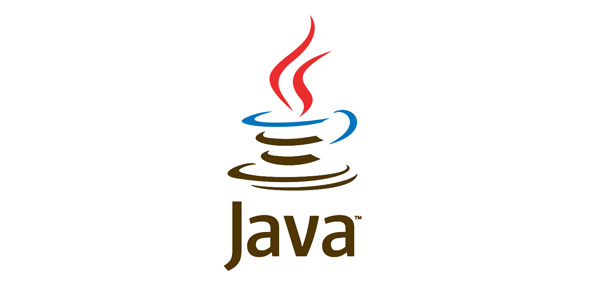# Fresher Drive @ 27th September Java

21 Questions | Attempts: 89
ShareSettings• 1.
What will be the output?public class Test {            static String  a;            static String  b;            public static void main(String ars[]){                        System.out.print(a+b);            }}
• A.

Null

• B.

Compile time error

• C.

Nullnull

• D.

0

• 2.
You want a class to have access to members of another class in the same package. Which is the most restrictive access that accomplishes this objective?
• A.

Public

• B.

Private

• C.

Protected

• D.

Default access

• 3.
Which is a valid declaration within an interface?
• A.

Public static short stop = 23

• B.

Protected short stop = 23

• C.

Transient short stop = 23

• D.

• 4.
What will be the output of the program?class Base{    Base()    {        System.out.print("Base");    }}public class Alpha extends Base{    public static void main(String[] args)    {        new Alpha();        new Base();    }}
• A.

Base

• B.

BaseBase

• C.

Compilation fails

• D.

The code runs with no output

• 5.
What will be the output of the program?class Equals    public static void main(String [] args)    {        int x = 100;        double y = 100.1;        boolean b = (x = y);        System.out.println(b);    }
• A.

True

• B.

False

• C.

Compilation fails

• D.

An exception is thrown at runtime

• 6.
What will be the output of the program?for(int i = 0; i < 3; i++){    switch(i)    {        case 0: break;        case 1: System.out.print("one ");        case 2: System.out.print("two ");        case 3: System.out.print("three ");    }System.out.printIn("done");
• A.

Done

• B.

One two done

• C.

One two three done

• D.

One two three two three done

• 7.
Which is a valid keyword in java?
• A.

Interface

• B.

String

• C.

Float

• D.

Unsigned

• 8.
Which method must be defined by a class implementing the java.lang.Runnable interface?
• A.

Void run()

• B.

Public void run()

• C.

Public void start()

• D.

Void run(int priority)

• 9.
Which interface does java.util.Hashtable implement?
• A.

Java.util.Map

• B.

Java.util.List

• C.

Java.util.HashTable

• D.

Java.util.Collection

• 10.
Which class cannot be a subclass in java?
• A.

Abstract class

• B.

Parent class

• C.

Final class

• D.

None of above

• 11.
Why we use array as a parameter of main method?
• A.

It is syntax

• B.

Can store multiple values

• C.

Both of above

• D.

None of above

• 12.
What will be the output of the program?public class Foo{     public static void main(String[] args)    {        try        {            return;        }        finally        {            System.out.println( "Finally" );        }    }}
• A.

Finally

• B.

Compilation fails

• C.

The code runs with no output

• D.

An exception is thrown at runtime

• 13.
Which statement is true about a static nested class?
• A.

You must have a reference to an instance of the enclosing class in order to instantiate it

• B.

It does not have access to nonstatic members of the enclosing class

• C.

It's variables and methods must be static

• D.

It must extend the enclosing class

• 14.
Which three are methods of the Object class?   notify();   notifyAll();   isInterrupted();   synchronized();   interrupt();   wait(long msecs);   sleep(long msecs);   yield();
• A.

1, 2, 4

• B.

2, 4, 5

• C.

1, 2, 6

• D.

2, 3, 4

• 15.
Which cause a compiler error?
• A.

Int[ ] scores = {3, 5, 7};

• B.

Int [ ][ ] scores = {2,7,6}, {9,3,45};

• C.

String cats[ ] = {"Fluffy", "Spot", "Zeus"};

• D.

Boolean results[ ] = new boolean [] {true, false, true};

• E.

Integer results[ ] = {new Integer(3), new Integer(5), new Integer(8)};

• 16.
Which method executes only once?
• A.

Start() method

• B.

Init() method

• C.

Stop() method

• D.

Destroy() method

• 17.
Which is a valid keyword in java?
• A.

Interface

• B.

String

• C.

Float

• D.

Unsigned

• 18.
Which class cannot be a subclass in java?
• A.

Abstract class

• B.

Parent class

• C.

Final class

• D.

None of above

• 19.
What will be the output of the program?public class Foo{     public static void main(String[] args)    {        try        {            return;        }        finally        {            System.out.println( "Finally" );        }    }}
• A.

Finally

• B.

Compilation fails

• C.

The code runs with no output

• D.

An exception is thrown at runtime

• 20.
Which statement is true about a static nested class?
• A.

You must have a reference to an instance of the enclosing class in order to instantiate it.

• B.

It does not have access to nonstatic members of the enclosing class

• C.

It's variables and methods must be static

• D.

It must extend the enclosing class

• 21.
Which cause a compiler error?
• A.

Int[ ] scores = {3, 5, 7};

• B.

Int [ ][ ] scores = {2,7,6}, {9,3,45};

• C.

String cats[ ] = {"Fluffy", "Spot", "Zeus"};

• D.

Boolean results[ ] = new boolean [] {true, false, true};

• E.

Integer results[ ] = {new Integer(3), new Integer(5), new Integer(8)};

## Related TopicsBack to top
×

Wait!
Here's an interesting quiz for you.